# 12 Results

View
Selected filters:
• BEDMAS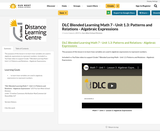Conditional Remix & Share Permitted
CC BY-NC-SA
Rating

The purpose of this lesson is to learn how variables are used in algebraic expressions to represent numbers.

Included is a YouTube video to support Grade 7 Blended Learning Math - Unit 1.3: Patterns and Relations - Algebraic Expressions.

Subject:
Math
Material Type:
Activity/Lab
Homework/Assignment
Lesson
Provider:
Sun West Distance Learning Centre (DLC)
Author:
Sun West School Division
06/06/2019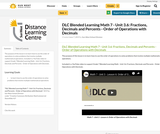Conditional Remix & Share Permitted
CC BY-NC-SA
Rating

The purpose of this lesson is to learn how to use the order of operations to solve problems that involve multiple mathematical operations.

Included is a YouTube video to support Grade 7 Blended Learning Math - Unit 3.6: Fractions, Decimals and Percents - Order of Operations with Decimals.

Subject:
Math
Material Type:
Activity/Lab
Homework/Assignment
Lesson
Provider:
Sun West Distance Learning Centre (DLC)
Author:
Sun West School Division
06/07/2019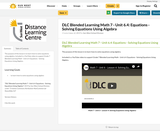Conditional Remix & Share Permitted
CC BY-NC-SA
Rating

The purpose of this lesson is to learn how to solve equations using algebra.

Included is a YouTube video to support Grade 7 Blended Learning Math - Unit 6.4: Equations - Solving Equations Using Algebra.

Subject:
Math
Material Type:
Activity/Lab
Homework/Assignment
Lesson
Provider:
Sun West Distance Learning Centre (DLC)
Author:
Sun West School Division
06/12/2019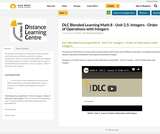Conditional Remix & Share Permitted
CC BY-NC-SA
Rating

The purpose of this lesson is to learn how to solve problems that include several different operations, including multiplication, division, subtraction and addition with negative and positive integers.

Included is a YouTube video to support Grade 8 Blended Learning Math - Unit 2.5: Integers - Order of Operations with Integers.

Subject:
Math
Material Type:
Activity/Lab
Homework/Assignment
Lesson
Provider:
Sun West Distance Learning Centre (DLC)
Author:
Sun West School Division
06/14/2019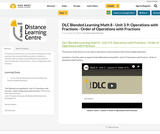Conditional Remix & Share Permitted
CC BY-NC-SA
Rating

The purpose of this lesson is to use order of operations to solve questions that involve multiple operations.

Included is a YouTube video to support Grade 8 Blended Learning Math - Unit 3.9: Operations with Fractions - Order of Operations with Fractions.

Subject:
Math
Material Type:
Activity/Lab
Homework/Assignment
Lesson
Provider:
Sun West Distance Learning Centre (DLC)
Author:
Sun West School Division
06/14/2019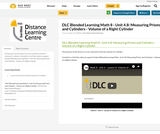Conditional Remix & Share Permitted
CC BY-NC-SA
Rating

The purpose of this lesson is to use a formula to find the volume of a cylinder.

Included is a YouTube video to support Grade 8 Blended Learning Math - Unit 4.8: Measuring Prisms and Cylinders - Volume of a Right Cylinder.

Subject:
Math
Material Type:
Activity/Lab
Homework/Assignment
Lesson
Provider:
Sun West Distance Learning Centre (DLC)
Author:
Sun West School Division
06/17/2019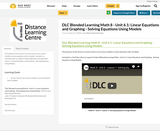Conditional Remix & Share Permitted
CC BY-NC-SA
Rating

The purpose of this lesson is to learn how to use various models to solve equations with variables.

Included is a YouTube video to support Grade 8 Blended Learning Math - Unit 6.1: Linear Equations and Graphing - Solving Equations Using Models.

Subject:
Math
Material Type:
Activity/Lab
Homework/Assignment
Lesson
Provider:
Sun West Distance Learning Centre (DLC)
Author:
Sun West School Division
06/17/2019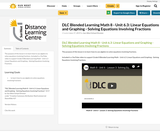Conditional Remix & Share Permitted
CC BY-NC-SA
Rating

The purpose of this lesson is to learn how to use algebra to solve equations involving fractions.

Included is a YouTube video to support Grade 8 Blended Learning Math - Unit 6.3: Linear Equations and Graphing - Solving Equations Involving Fractions.

Subject:
Math
Material Type:
Activity/Lab
Homework/Assignment
Lesson
Provider:
Sun West Distance Learning Centre (DLC)
Author:
Sun West School Division
06/17/2019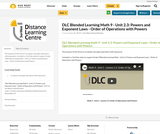Conditional Remix & Share Permitted
CC BY-NC-SA
Rating

The purpose of this lesson is to explain and apply operations with exponents.

Included is a YouTube video to support Grade 9 Blended Learning Math - Unit 2.3: Powers and Exponent Laws - Order of Operations with Powers.

Subject:
Math
Material Type:
Activity/Lab
Homework/Assignment
Lesson
Provider:
Sun West Distance Learning Centre (DLC)
Author:
Sun West School Division
06/18/2019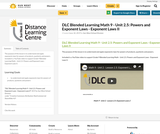Conditional Remix & Share Permitted
CC BY-NC-SA
Rating

The purpose of this lesson is to understand and apply exponents laws for powers of products, quotients and powers.

Included is a YouTube video to support Grade 9 Blended Learning Math - Unit 2.5: Powers and Exponent Laws - Exponent Laws II.

Subject:
Math
Material Type:
Activity/Lab
Homework/Assignment
Lesson
Provider:
Sun West Distance Learning Centre (DLC)
Author:
Sun West School Division
06/18/2019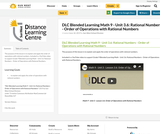Conditional Remix & Share Permitted
CC BY-NC-SA
Rating

The purpose of this lesson is to explain and apply the order of operations with rational numbers.

Included is a YouTube video to support Grade 9 Blended Learning Math - Unit 3.6: Rational Numbers - Order of Operations with Rational Numbers.

Subject:
Math
Material Type:
Activity/Lab
Homework/Assignment
Lesson
Provider:
Sun West Distance Learning Centre (DLC)
Author:
Sun West School Division
06/18/2019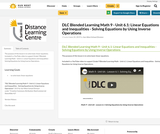Conditional Remix & Share Permitted
CC BY-NC-SA
Rating

The purpose of this lesson is to solve basic linear equations.

Included is a YouTube video to support Grade 9 Blended Learning Math - Unit 6.1: Linear Equations and Inequalities - Solving Equations by Using Inverse Operations.

Subject:
Math
Material Type:
Activity/Lab
Homework/Assignment
Lesson
Provider:
Sun West Distance Learning Centre (DLC)
Author:
Sun West School Division